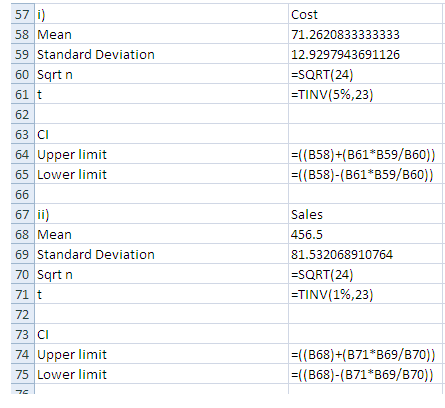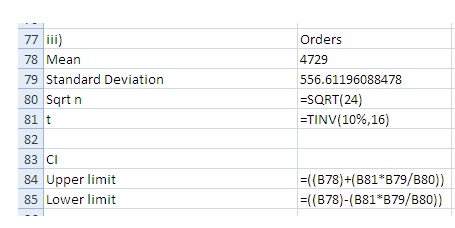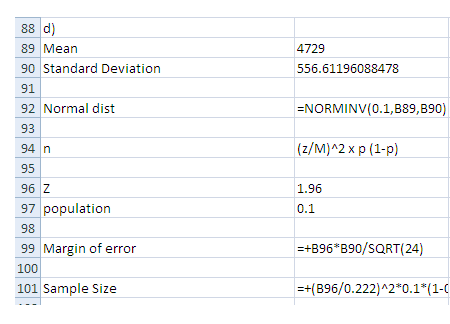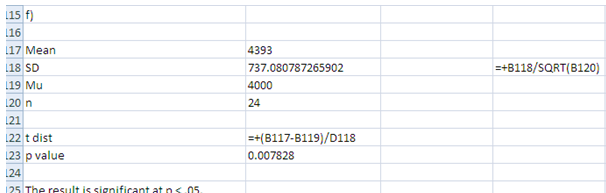## Part A

### Mean and Standard deviation

 Cost Sales Orders 52.95 386 4015 71.66 446 3806 85.58 512 5309 63.69 401 4262 72.81 457 4296 68.44 458 4097 52.46 301 3213 70.77 484 4809 82.03 517 5237 74.39 503 4732 70.84 535 4413 54.08 353 2921 62.98 372 3977 72.3 328 4428 58.99 408 3964 79.38 491 4582 94.44 527 5582 59.74 444 3450 90.5 623 5079 93.24 596 5735 69.33 463 4269 53.71 389 3708 89.18 547 5387 66.8 415 4161
 Cost Sales Orders Mean 71.26 456.50 4,393.00 Standard Deviation 12.93 81.53 737.08

### Question b

Standard deviation of all orders above 4,000

Sample proportion

 Orders above 4000 4015 5309 4262 4296 4097 4809 5237 4732 4413 4428 4582 5582 5079 5735 4269 5387 4161
 Mean 4,729.00 Standard deviation 556.61

Question c

Confidence intervals

95% for distribution costSince the total value is less than 30, t distribution is used to measure the confidence interval

 i) Cost Mean 71.26 Standard Deviation 12.93 Sqrt n 4.90 t 2.07
 CI Upper limit 76.72 Lower limit 65.80

99% confidence interva on sales

 ii) Sales Mean 456.50 Standard Deviation 81.53 Sqrt n 4.90 t 2.81 CI Upper limit 503.22 Lower limit 409.78

90% confidence interval on orders exceed 4000

 iii) Orders Mean 4,729.00 Standard Deviation 556.61 Sqrt n 4.90 t 1.75 CI Upper limit 4,927.36 Lower limit 4,530.64

### Question d

Point estimate

 Mean 4,729.00 Standard Deviation 556.61 Normal dist 4,015.67

N = (z/M)^2 x p (1-p)

 Z 1.96 population 0.1 Margin of error 222.69 Sample Size 7.02

### Question e

Null hypothesis: The mean is equal to 65

Alternate hypothesis: The mean is not equal to 65

 x 71.26 Standard Deviation 12.93 Mu 65 n 24 t dist 2.37 t table value 2.07

Since t distribution is greater than t table value the null hypothesis is rejected and alternate hypithesis is accepted

### Question f

Null hypothesis: 30% orders received are less than 4000

Alternate hypothesis: 30% orders received are not less than 4000

 Mean 4,393.00 SD 737.08 Mu 4000 n 24 t dist 2.61 p value 0.007828

The result is significant at p < .05.

### Question g

 Cost 52.95 71.66 85.58 63.69 72.81 68.44 52.46 70.77 82.03 74.39 70.84 54.08

Null hypothesis: The mean is equal to 65

Alternate hypothesis: The mean is not equal to 65

 Mean 68.31 SD 10.78 Mu 65 n 12 t dist 1.06 t table value 3.11

Since t distribution is less than t table value the null hypothesis is accepted

### Question a### Question b### Question c### Question ePart C

### Question a

 Cost and Sales SUMMARY OUTPUT Regression Statistics Multiple R 0.842117773 R Square 0.709162344 Adjusted R Square 0.69594245 Standard Error 7.129671393 Observations 24 ANOVA df SS MS F Significance F Regression 1 2726.821684 2726.821684 53.64357481 2.47416E-07 Residual 22 1118.308712 50.83221418 Total 23 3845.130396 Coefficients Standard Error t Stat P-value Lower 95% Upper 95% Lower 95.0% Upper 95.0% Intercept 10.29761784 8.449998094 1.218653274 0.235882952 -7.226605545 27.82184123 -7.226605545 27.82184123 Sales 0.13354757 0.018233798 7.324177415 2.47416E-07 0.095732988 0.171362151 0.095732988 0.171362151
 Cost and Orders SUMMARY OUTPUT Regression Statistics Multiple R 0.91880399 R Square 0.844200772 Adjusted R Square 0.837118989 Standard Error 5.218273602 Observations 24 ANOVA df SS MS F Significance F Regression 1 3246.062049 3246.062049 119.2073751 2.38511E-10 Residual 22 599.0683465 27.23037939 Total 23 3845.130396 Coefficients Standard Error t Stat P-value Lower 95% Upper 95% Lower 95.0% Upper 95.0% Intercept 0.457625305 6.571882688 0.069633821 0.945114194 -13.17162514 14.08687576 -13.17162514 14.08687576 Orders 0.016117564 0.001476209 10.918213 2.38511E-10 0.013056094 0.019179034 0.013056094 0.019179034

Based on the above outputs it is identified that the value of R square betwee cost and sales is 0.7091 or 70.91% whereas the value is 0.8442 or 84.42% for cost and orders. The value of R square intends to specify the goodness of the fit of the model, the maximum value of R square is 1 or 100%, so higher the value of R square better is the goodness of fit to the model. So it can be stated that cost and orders shows a better association in the model

### Question c

Multiple regression

 Cost - Sales and Orders SUMMARY OUTPUT Regression Statistics Multiple R 0.93591442 R Square 0.875935802 Adjusted R Square 0.864120164 Standard Error 4.766165573 Observations 24 ANOVA df SS MS F Significance F Regression 2 3368.087376 1684.043688 74.1336022 3.0429E-10 Residual 21 477.0430196 22.71633427 Total 23 3845.130396 Coefficients Standard Error t Stat P-value Lower 95% Upper 95% Lower 95.0% Upper 95.0% Intercept -2.728246583 6.157879754 -0.443049668 0.662260247 -15.53425853 10.07776536 -15.53425853 10.07776536 Sales 0.047113872 0.02032792 2.317692762 0.030643769 0.004839649 0.089388095 0.004839649 0.089388095 Orders 0.011946926 0.002248569 5.313123092 2.87239E-05 0.00727077 0.016623082 0.00727077 0.016623082

By using multiple regression where in the sales and orders are considered as independent variables ad the dependent variable is cost. The model states that the R square is 0.8759 or 87.59% which is a better fit when compared with individual models which was specified in question a. Also the significance value is 0.00 which shows that there is a significant relationship between the independent variables and the dependent variable.

Based on the regression coefficients the regression equation can be stated as

Y (Cost) = Constant + X1 (Sales) + X2 (Orders)

Y (Cost) = -2.73 + 0.047 (Sales) + 0.0119 (Orders)

Based on the above it is noted that orders possess a significant influence on the cost when compared with sales

### Question a

F test

Null hypothesis: There is no significant variance of the waiting time between the two branches

Alternate hypothesis: There is a significant variance of the waiting time between the two branches

 CBD Suburban Mean 4.286666667 6.873571429 Variance 2.682995238 3.73004011 Observations 15 14 df 14 13 F 0.719293938 P(F<=f) one-tail 0.274097371 F Critical one-tail 0.398841227

Based on the above table it is identified that the p value is 0.274 which is more than the sig value of 0.05 (5%) so the null hypothesis is accepted. Hence it is stated that There is no significant variance of the waiting time between the two branches

### Question b

Based on the overall analysis it is identified that the t-Test: Paired Two Sample for Means can be used

Question c

Null hypothesis: There is no difference in the mean waiting time between the two branches

Alternatehypothesis: There is a difference in the mean waiting time between the two branches

 CBD Suburban Mean 4.286666667 7.114666667 Variance 2.682995238 4.335512381 Observations 15 15 Pearson Correlation 0.176721009 Hypothesized Mean Difference 0 df 14 t Stat -4.542789694 P(T<=t) one-tail 0.000229993 t Critical one-tail 1.761310136 P(T<=t) two-tail 0.000459985 t Critical two-tail 2.144786688

Based on the above table it is identified that the p-value is 0.00 which is less than the sig value of 0.05 (5%) so the null hypothesis is rejected and alternate hypothesis is accepted. Hence, There is a difference in the mean waiting time between the two branches.

#### 1. Boslaugh, Sarah (2012). Statistics in a Nutshell. Cengage Publishing

2. Freedman, David (2010). Statistics. 4th Edition. Cengage Publishing

3. Sincich, T. Terry (2012). Statistics. 12th Edition.

4. Triola (2014). Essentials of Statistics. 5th Edition. McGraw Hill

5. Witte, S. Robert. (2010). Statistics. 5th Edition. McGraw Hill